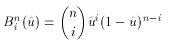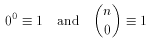# glMap2

### NAME

glMap2d, glMap2f -- define a two-dimensional evaluator

### C SPECIFICATION

void glMap2d(GLenum target,
GLdouble u1,
GLdouble u2,
GLint ustride,
GLint uorder,
GLdouble v1,
GLdouble v2,
GLint vstride,
GLint vorder,
const GLdouble *points)
void glMap2f(GLenum target,
GLdouble u1,
GLdouble u2,
GLint ustride,
GLint uorder,
GLdouble v1,
GLdouble v2,
GLint vstride,
GLint vorder,
const GLfloat *points)


### PARAMETERS

target
Specifies the kind of values that are generated by the evaluator. Symbolic constants GL_MAP2_VERTEX_3, GL_MAP2_VERTEX_4, GL_MAP2_INDEX, GL_MAP2_COLOR_4, GL_MAP2_NORMAL, GL_MAP2_TEXTURE_COORD_1, GL_MAP2_TEXTURE_COORD_2, GL_MAP2_TEXTURE_COORD_3, and GL_MAP2_TEXTURE_COORD_4 are accepted.

u1, u2
Specify a linear mapping of u, as presented to glEvalCoord2, to u^, one of the two variables that is evaluated by the equations specified by this command.

ustride
Specifies the number of floats or doubles between the beginning of control point Rij and the beginning of control point R(i+1)j, where i and j are the u and v control point indices, respectively. This allows control points to be embedded in arbitrary data structures. The only constraint is that the values for a particular control point must occupy contiguous memory locations.

uorder
Specifies the dimension of the control point array in the u axis. Must be positive.

v1, v2
Specify a linear mapping of v, as presented to glEvalCoord2, to v^, one of the two variables that is evaluated by the equations specified by this command.

vstride
Specifies the number of floats or doubles between the beginning of control point Rij and the beginning of control point Ri(j+1), where i and j are the u and v control point indices, respectively. This allows control points to be embedded in arbitrary data structures. The only constraint is that the values for a particular control point must occupy contiguous memory locations.

vorder
Specifies the dimension of the control point array in the v axis. Must be positive.

points
Specifies a pointer to the array of control points.

### DESCRIPTION

Evaluators provide a way to use polynomial or rational polynomial mapping to produce vertices, normals, texture coordinates, and colors. The values produced by an evaluator are sent on to further stages of GL processing just as if they had been presented using glVertex, glNormal, glTexCoord, and glColor commands, except that the generated values do not update the current normal, texture coordinates, or color.

All polynomial or rational polynomial splines of any degree (up to the maximum degree supported by the GL implementation) can be described using evaluators. These include almost all surfaces used in computer graphics, including B-spline surfaces, NURBS surfaces, Bezier surfaces, and so on.

Evaluators define surfaces based on bivariate Bernstein polynomials. Define p(u^,v^) aswhere Rij is a control point, Bni(u^) is the ith Bernstein polynomial of degree n (uorder = n + 1)and Bmj(v^) is the jth Bernstein polynomial of degree m (vorder = m + 1)Recall thatglMap2 is used to define the basis and to specify what kind of values are produced. Once defined, a map can be enabled and disabled by calling glEnable and glDisable with the map name, one of the nine predefined values for target, described below. When glEvalCoord2 presents values u and v, the bivariate Bernstein polynomials are evaluated using u^ and v^, wheretarget is a symbolic constant that indicates what kind of control points are provided in points, and what output is generated when the map is evaluated. It can assume one of nine predefined values:

GL_MAP2_VERTEX_3
Each control point is three floating-point values representing x, y, and z. Internal glVertex3 commands are generated when the map is evaluated.

GL_MAP2_VERTEX_4
Each control point is four floating-point values representing x, y, z, and w. Internal glVertex4 commands are generated when the map is evaluated.

GL_MAP2_INDEX
Each control point is a single floating-point value representing a color index. Internal glIndex commands are generated when the map is evaluated. The current index is not updated with the value of these glIndex commands, however.

GL_MAP2_COLOR_4
Each control point is four floating-point values representing red, green, blue, and alpha. Internal glColor4 commands are generated when the map is evaluated. The current color is not updated with the value of these glColor4 commands however.

GL_MAP2_NORMAL
Each control point is three floating-point values representing the x, y, and z components of a normal vector. Internal glNormal commands are generated when the map is evaluated. The current normal is not updated with the value of these glNormal commands, however.

GL_MAP2_TEXTURE_COORD_1
Each control point is a single floating-point value representing the s texture coordinate. Internal glTexCoord1 commands are generated when the map is evaluated. The current texture coordinates are not updated with the value of these glTexCoord1 commands, however.

GL_MAP2_TEXTURE_COORD_2
Each control point is a single floating-point value representing the s and t texture coordinates. Internal glTexCoord2 commands are generated when the map is evaluated. The current texture coordinates are not updated with the value of these glTexCoord commands, however.

GL_MAP2_TEXTURE_COORD_3
Each control point is a single floating-point value representing the s, t and r texture coordinates. Internal glTexCoord3 commands are generated when the map is evaluated. The current texture coordinates are not updated with the value of these glTexCoord commands, however.

GL_MAP2_TEXTURE_COORD_4
Each control point is a single floating-point value representing the s, t, r and q texture coordinates. Internal glTexCoord4 commands are generated when the map is evaluated. The current texture coordinates are not updated with the value of these glTexCoord commands, however.
ustride, uorder, vstride, vorder, and points define the array addressing for accessing the control points. points is the location of the first control point, which occupies one, two, three, or four contiguous memory locations, depending on which map is being defined. There are uorder * vorder control points in the array. ustride tells how many float or double locations are skipped to advance the internal memory pointer from control point Rij to control point R(i+1)j. vstride tells how many float or double locations are skipped to advance the internal memory pointer from control point Rij to control point Ri(j+1).

### NOTES

As is the case with all GL commands that accept pointers to data, it is as if the contents of points were copied by glMap2 before it returned. Changes to the contents of points have no effect after glMap2 is called.

### ERRORS

GL_INVALID_ENUM is generated if target is not an accepted value.

GL_INVALID_VALUE is generated if u1 is equal to u2, or if v1is equal to v2.

GL_INVALID_VALUE is generated if either ustride or vstride is less than the number of values in a control point.

GL_INVALID_VALUE is generated if either uorder or vorder is less than one or greater than GL_MAX_VAL_ORDER.

GL_INVALID_OPERATION is generated if glMap2 is called between a call to glBegin and the corresponding call to glEnd.

### ASSOCIATED GETS

glGetMap
glGet with argument GL_MAX_VAL_ORDER
glIsEnabled with argument GL_MAP2_VERTEX_3
glIsEnabled with argument GL_MAP2_VERTEX_4
glIsEnabled with argument GL_MAP2_INDEX
glIsEnabled with argument GL_MAP2_COLOR_4
glIsEnabled with argument GL_MAP2_NORMAL
glIsEnabled with argument GL_MAP2_TEXTURE_COORD_1
glIsEnabled with argument GL_MAP2_TEXTURE_COORD_2
glIsEnabled with argument GL_MAP2_TEXTURE_COORD_3
glIsEnabled with argument GL_MAP2_TEXTURE_COORD_4